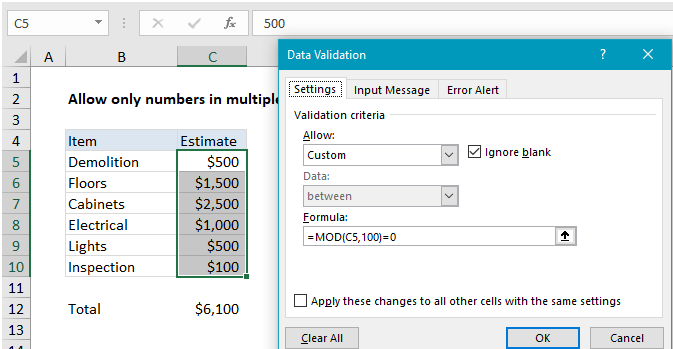## Excel Office

Excel How Tos, Tutorials, Tips & Tricks, Shortcuts

# Accept only numbers that are multiple of 100

To allow only numbers in multiples of 100, you can use data validation with a custom formula based on the MOD function.

## Formula

`=MOD(A1,100)=0`

##Note: Cell references in data validation formulas are relative to the upper left cell in the range selected when the validation rule is defined, in this case C5.

Also See:   MOD function: Description, Usage, Syntax, Examples and Explanation

## Explanation

In the example shown, the data validation applied to C5:C10 is:

`=MOD(C5,100)=0`

### How this formula works

Data validation rules are triggered when a user adds or changes a cell value. When a custom formula returns TRUE, validation passes and the input is accepted. When the formula returns FALSE, validation fails and the input is rejected.

Also See:   Basic timesheet formula with breaks in Excel

In this case, the MOD function is used to perform a modulo operation, which returns the remainder after division. The formula used to validate input is:

`=MOD(C5,100)=0`

The value in C5 is 500. The MOD function divides 500 by 100 and gets 5, with a remainder of zero. Since 0 = 0,  The rule returns TRUE and the data validation passes:

```=MOD(500,100)=0
=0=0
=TRUE```

If a user enters, say, 550, the remainder is 50, and validation fails:

```=MOD(C5,100)=0
=MOD(550,100)=0
=50=0
=FALSE```
Also See:   Convert time to time zone in Excel